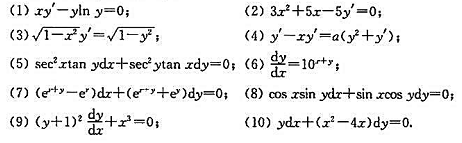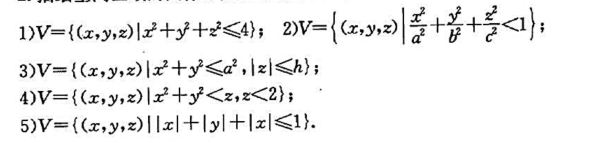(1)x2-y2=C,y|x=D=5;

(2)y=(C+C2x)e2x,y|x=D=0,y|x=D=1;

(3)y=C1sin(x -C2),y|x-π=1,y'|x-π=0.参考答案d(D)=sup{||P-Q|||P∈D,Q∈D}1477379517

¥49.8
0.54元/天
3个月
¥39.8
¥60.00
1个月
¥99.8
¥150.00
1年微信付款支付宝付款